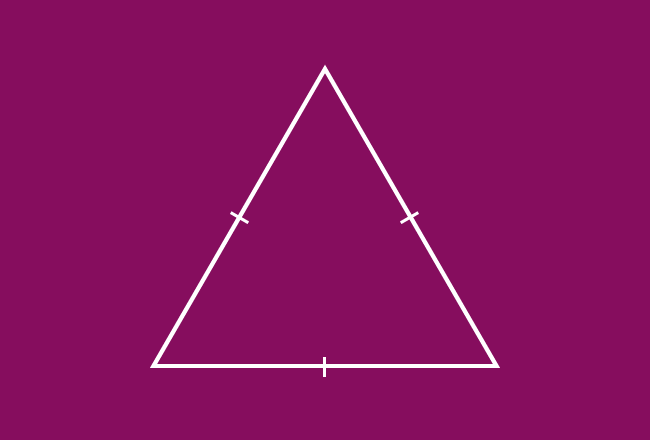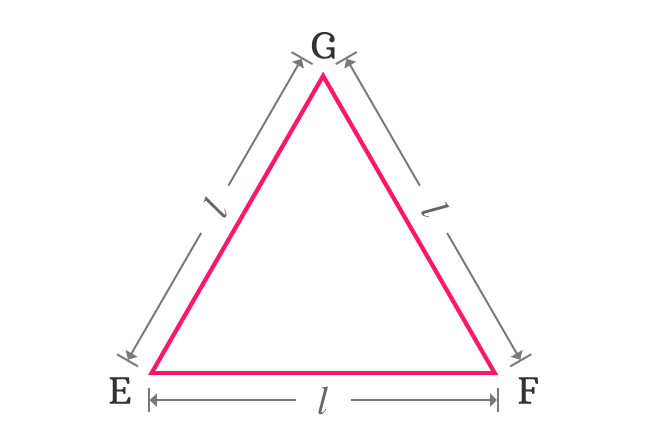# Equilateral triangle

A triangle whose lengths of all three sides are equal is called an equilateral triangle.

## IntroductionA triangle can be constructed by connecting endpoints of three line segments of same length as a closed geometric shape. Actually, the meaning of equilateral is sides of the same length. So, the triangle is called as an equilateral triangle.

The lengths of all three sides of an equilateral triangle are equal due to the construction of triangle with the line segments which have same length. Due to same reason, its interior angles are also equal and each angle is equal to $60^°$. Hence, it is also called as an equiangular triangle.

#### Example

$\Delta EFG$ is a triangle and length of each side is $l$. So, this triangle is called as an equilateral triangle.### Properties

The lengths of all three sides of $\Delta EFG$ are equal.

$EF = FG = GE = l$

It is an equiangular triangle. So, all three angles of this triangle are equal and each angle is $60^°$.

$\angle EFG$ $\,=\,$ $\angle FGE$ $\,=\,$ $\angle GEF$ $\,=\,$ $60^°$

### Construction

Learn how to construct an equilateral triangle geometrically by using geometric tools.

A best free mathematics education website that helps students, teachers and researchers.

###### Maths Topics

Learn each topic of the mathematics easily with understandable proofs and visual animation graphics.

###### Maths Problems

A math help place with list of solved problems with answers and worksheets on every concept for your practice.

Learn solutions

###### Subscribe us

You can get the latest updates from us by following to our official page of Math Doubts in one of your favourite social media sites.